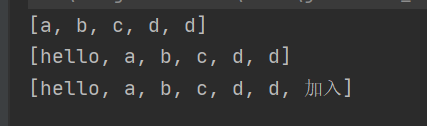﻿ Java中关于泛型、包装类及ArrayList的详细教程_java_脚本之家
java# Java中关于泛型、包装类及ArrayList的详细教程

## 一、泛型

### 1.1 泛型类的定义

```// 1. 尖括号 <> 是泛型的标志
// 2. E 是类型变量(Type Variable)，变量名一般要大写
// 3. E 在定义时是形参，代表的意思是 MyArrayList 最终传入的类型，但现在还不知道
public class MyArrayList<E> {
private E[] array;
private int size;
}
```

1. 泛型是作用在编译期间的一种机制，即运行期间没有泛型的概念
2. 泛型代码在运行期间，利用 Object 达到的效果（这里不是很准确）。
3. 泛型的意义：自动对类型进行检查

### 1.2 泛型类的使用

```class MyArrayList<E>{//  <E>代表当前类是一个泛型类，此时的E就是一个占位符而已
private E[] elem;
private int usedSize;

public MyArrayList() {
//        this.elem = elem;
this.elem = (E[])new Object;//这样的写法不是十分正确
}
this.elem[usedSize] = val;
usedSize++;
}
public E get(int pos){
return this.elem[pos];
}
}
public class test02 {
public static void main(String[] args) {
//泛型中尖括号里面的内容不参与类型的组成
MyArrayList<String>  myArrayList = new MyArrayList<>();
System.out.println(myArrayList);
MyArrayList<Integer>  myArrayList1 = new MyArrayList<>();
System.out.println(myArrayList1);
MyArrayList<Boolean>  myArrayList2 = new MyArrayList<>();
System.out.println(myArrayList2);
}
public static void main2(String[] args) {
MyArrayList<String>  myArrayList = new MyArrayList<>();
String ret = myArrayList.get(1);//自动对类型进行强制类型转换
System.out.println(ret);
}
}
```

### 1.3 泛型总结

1. 泛型是为了解决某些容器、算法等代码的通用性而引入，并且能在编译期间做类型检查。
2. 泛型利用的是 `Object` 是所有类的祖先类，并且父类的引用可以指向子类对象的特定而工作。
3. 泛型是一种编译期间的机制，即 `MyArrayList<Integer>``MyArrayList<String>` 在运行期间是一个类型。即泛型中尖括号里面的内容不参与类型的组成。
4. 泛型是 `java` 中的一种合法语法，标志就是尖括号 `<>`

## 二、包装类

byte Byte
short Short
int Integer
long Long
float Float
double Double
char Character
boolean Boolean

### 2.2 包装类的使用，装箱(boxing)和拆箱(unboxing)

```public static void main(String[] args) {
Integer a = 123;//装箱 装包（隐式的）
//Integer 的范围[-128,127];
int b= a;//拆箱 拆包（隐式的）
System.out.println("a="+a+" "+ "b="+ b);
System.out.println("===============");
Integer a2 = Integer.valueOf(123);//显式的装包
Integer a3 = new Integer(123);//显式的装包

int b2 = a2.intValue();//显式的拆包
double d = a2.doubleValue();//显式的拆包
int i = 10;//显式的初始化
}
```

### 2.3 自动装箱(autoboxing)和自动拆箱(autounboxing)

```int b2 = a2.intValue();//显式的拆包
double d = a2.doubleValue();//显式的拆包
```

## 三、List 的使用

### 3.1 ArrayList简介• `ArrayList`实现了`RandomAccess`接口，表明`ArrayList`支持随机访问
• `ArrayList`实现了`Cloneable`接口，表明`ArrayList`是可以`clone`
• `ArrayList`实现了`Serializable`接口，表明`ArrayList`支持序列化的
• `Vector`不同，`ArrayList`不是线程安全的，在单线程下可以使用，在多线程中可以选择Vector或者`CopyOnWriteArrayList`
• `ArrayList`底层是一段连续的空间，并且可以动态扩容，是一个动态类型的顺序表。 3.2 ArrayList的构造
```public static void main(String[] args) {
// ArrayList创建，推荐写法
// 构造一个空的列表
List<Integer> list1 = new ArrayList<>();
// 构造一个具有10个容量的列表
List<Integer> list2 = new ArrayList<>(10);
// list3构造好之后，与list2中的元素一致
//使用另外一个ArrayList对list3进行初始化
ArrayList<String> list3 = new ArrayList<>(list2);
// 避免省略类型，否则：任意类型的元素都可以存放，使用时将是一场灾难
List list4 = new ArrayList();
}
```

### 3.3 ArrayList的遍历

`ArrayList` 可以使用三种方式遍历：`for循环+下标``foreach``使用迭代器`

``` public static void main(String[] args) {
List<String> list = new ArrayList<>();
ArrayList<String> list2 = new ArrayList<>();
System.out.println(list2);//打印方式一
System.out.println("============");
for (int i = 0; i < list2.size(); i++) {//打印方式二
System.out.print(list2.get(i)+" ");
}
System.out.println();
System.out.println("==========");
for (String s:list2) {//打印方式三
System.out.print(s+" ");
}
System.out.println();
System.out.println("====迭代器打印方式1======");
Iterator<String> it = list2.iterator();
while (it.hasNext()){
System.out.print(it.next()+" ");
}
System.out.println();
System.out.println("=====迭代器打印方式2=====");
ListIterator<String> it2 = list2.listIterator();//打印方式四，使用迭代器进行打印
while (it2.hasNext()){
System.out.print(it2.next()+" ");
}
}
```### 3.4 ArrayList的常见操作

#### 3.4.1 删除 index 位置元素(remove)

```E remove(int index) //删除 index 位置元素
```

``` public static void main(String[] args) {
ArrayList<String> list2 = new ArrayList<>();
Iterator<String> it = list2.iterator();
while (it.hasNext()) {
String ret = it.next();
if(ret.equals("hello")) {
it.remove();//首先需要使用next方法迭代出集合中的元素 ，然后才能调用remove方法
}else {
System.out.print(ret + " ");
}
}

System.out.println();
System.out.println("========迭代器List相关打印==========");
ListIterator<String> it2 = list2.listIterator();
while (it2.hasNext()) {
String ret = it2.next();
if(ret.equals("hello")) {
it2.remove();//首先需要使用next方法迭代出集合中的元素 ，然后才能调用remove方法
}else {
System.out.print(ret + " ");
}
}
}
``````boolean add(E e) //尾插 e
```

``` public static void main(String[] args) {
ArrayList<String> list2 = new ArrayList<>();
//CopyOnWriteArrayList<String> list2 = new CopyOnWriteArrayList<>();

/* Iterator<String> it = list2.iterator();
while (it.hasNext()) {
String ret = it.next();
if(ret.equals("hello")) {
}else {
System.out.print(ret + " ");
}
}*/

//使用ListIterator迭代器的方法添加元素，会将元素添加到其紧跟着的后面
ListIterator<String> it2 = list2.listIterator();
while (it2.hasNext()) {
String ret = it2.next();
if(ret.equals("bit")) {
//但是将上面的list2变成CopyOnWriteArrayList<String>类型就不会报错。
}else {
System.out.print(ret + " ");
}
}
System.out.println("=================");
System.out.println(list2);
}
``````  ArrayList<String> list2 = new ArrayList<>();//不是线程安全的
CopyOnWriteArrayList<String> list2 = new CopyOnWriteArrayList<>();//是线程安全的
```

```void add(int index, E element) //将 e 插入到 index 位置
boolean addAll(Collection<? extends E> c) //尾插 c 中的元素
```

```public static void main(String[] args) {
ArrayList<String> list2 = new ArrayList<>();
System.out.println(list2);
ArrayList<String> list3 = new ArrayList<>();
System.out.println(list2);
}
```#### 3.4.7 将指定下标位置的元素设置为指定值set()

```E remove(int index) //删除 index 位置元素
boolean remove(Object o) //删除遇到的第一个 o
E get(int index) //获取下标 index 位置元素
E set(int index, E element) //将下标 index 位置元素设置为 element
```

``` public static void main(String[] args) {
ArrayList<String> list2 = new ArrayList<>();
String ret = list2.remove(0);//删除0下标位置元素
System.out.println(list2);

boolean ret1 = list2.remove("d"); //删除遇到的第一个"d"
//如果要删除的数list2中没有则返回false
System.out.println(ret1); //true
String ret2 = list2.get(2);//获取下标为2位置的元素
System.out.println(ret2);//d
String ret3 = list2.set(2,"hello");//将下标为2位置的元素改为"hello"
System.out.println(ret3);//d
System.out.println(list2);//[b, c, hello]
```#### 3.4.12 截取部分 list 中的部分元素 subList()

```void clear() //清空
boolean contains(Object o) //判断 o是否在线性表中
int indexOf(Object o) //返回第一个 o 所在下标
int lastIndexOf(Object o) //返回最后一个 o 的下标
List<E> subList(int fromIndex, int toIndex) //截取部分 list
```

``` public static void main(String[] args) {
ArrayList<String> list2 = new ArrayList<>();
System.out.println(list2.indexOf("a"));//0 判断a的下标位置
System.out.println(list2.lastIndexOf("d"));//4 判断最后一个d出现的下标位置
List<String> sub = list2.subList(1,3);//[b, c] 截取下标为[1,3）位置的元素，截取的区间范围为左闭右开的
System.out.println(sub);//[b, c]
System.out.println(list2);//[a, b, c, d, d]
System.out.println("===================");
sub.set(0,"p");
System.out.println(sub);//[p, c]
System.out.println(list2);//[a, p, c, d, d]
System.out.println(list2.contains("c"));//true //判断list2当中是否包含字符"c"
list2.clear();//将list2中的元素清空
System.out.println(list2);
```